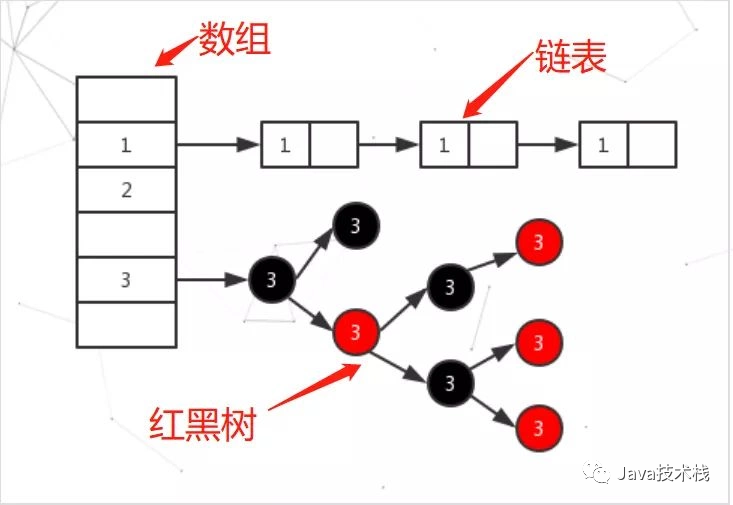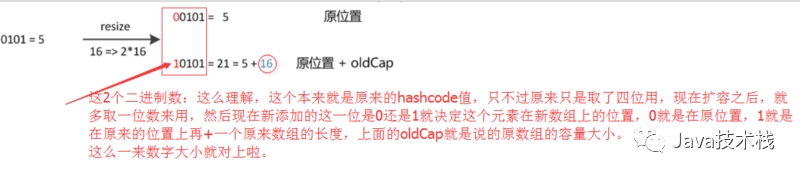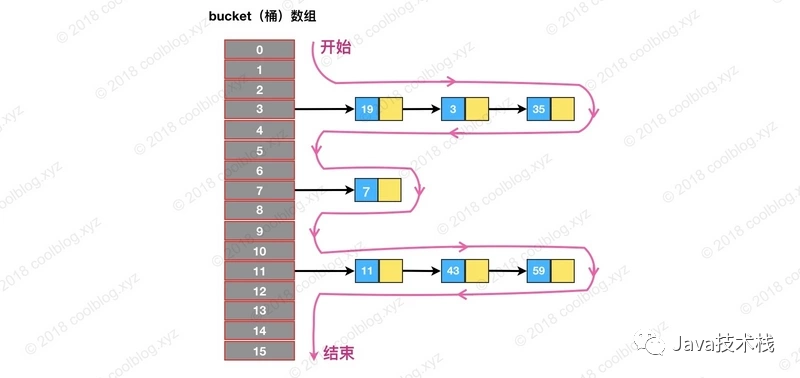•  默认大小、负载因子以及扩容倍数是多少

•  底层数据结构

•  如何处理 hash 冲突的

•  如何计算一个 key 的 hash 值

•  数组长度为什么是 2 的幂次方

•  扩容、查找过程

•  在 JDK1.8 中，HashMap 是由数组+链表+红黑树构成

•  当一个值中要存储到 HashMap 中的时候会根据 Key 的值来计算出他的 hash，通过 hash 值来确认存放到数组中的位置，如果发生 hash 冲突就以链表的形式存储，当链表过长的话，HashMap 会把这个链表转换成红黑树来存储。//默认初始容量为16
static final int DEFAULT_INITIAL_CAPACITY = 1 << 4;
//默认负载因子为0.75
static final float DEFAULT_LOAD_FACTOR = 0.75f;
//Hash数组(在resize()中初始化)
transient Node<K,V>[] table;
//元素个数
transient int size;
//容量阈值(元素个数超过该值会自动扩容)
int threshold;


table 数组里面存放的是 Node 对象，Node 是 HashMap 的一个内部类，用来表示一个 key-value，源码如下：

static class Node<K,V> implements Map.Entry<K,V> {
final int hash;
final K key;
V value;
Node<K,V> next;

Node(int hash, K key, V value, Node<K,V> next) {
this.hash = hash;
this.key = key;
this.value = value;
this.next = next;
}

public final K getKey()        { return key; }
public final V getValue()      { return value; }
public final String toString() { return key + "=" + value; }
public final int hashCode() {
return Objects.hashCode(key) ^ Objects.hashCode(value);//^表示相同返回0，不同返回1
//Objects.hashCode(o)————>return o != null ? o.hashCode() : 0;
}

public final V setValue(V newValue) {
V oldValue = value;
value = newValue;
return oldValue;
}

public final boolean equals(Object o) {
if (o == this)
return true;
if (o instanceof Map.Entry) {
Map.Entry<?,?> e = (Map.Entry<?,?>)o;
//Objects.equals(1,b)————> return (a == b) || (a != null && a.equals(b));
if (Objects.equals(key, e.getKey()) && Objects.equals(value, e.getValue()))
return true;
}
return false;
}
}


•  默认初始容量为 16，默认负载因子为 0.75

•  threshold = 数组长度 * loadFactor，当元素个数超过threshold(容量阈值)时，HashMap 会进行扩容操作

•  table 数组中存放指向链表的引用

table 数组长度永远为 2 的幂次方

/*找到大于或等于 cap 的最小2的幂，用来做容量阈值*/
static final int tableSizeFor(int cap) {
int n = cap - 1;
n |= n >>> 1;
n |= n >>> 2;
n |= n >>> 4;
n |= n >>> 8;
n |= n >>> 16;
return (n < 0) ? 1 : (n >= MAXIMUM_CAPACITY) ? MAXIMUM_CAPACITY : n + 1;
}


tableSizeFor 的功能（不考虑大于最大容量的情况）是返回大于等于输入参数且最近的 2 的整数次幂的数。比如 10，则返回 16。

/*传入初始容量和负载因子*/
public HashMap(int initialCapacity, float loadFactor) {

if (initialCapacity < 0)
throw new IllegalArgumentException("Illegal initial capacity: " +initialCapacity);
if (initialCapacity > MAXIMUM_CAPACITY)
initialCapacity = MAXIMUM_CAPACITY;
throw new IllegalArgumentException("Illegal load factor: " +loadFactor);

this.threshold = tableSizeFor(initialCapacity);
}

##### 那么为什么要把数组长度设计为 2 的幂次方呢？

1. 当数组长度为 2 的幂次方时，可以使用位运算来计算元素在数组中的下标

HashMap 是通过 index=hash&(table.length-1) 这条公式来计算元素在 table 数组中存放的下标，就是把元素的 hash 值和数组长度减1的值做一个与运算，即可求出该元素在数组中的下标，这条公式其实等价于 hash%length，也就是对数组长度求模取余，只不过只有当数组长度为 2 的幂次方时，hash&(length-1) 才等价于 hash%length，使用位运算可以提高效率。

2. 增加 hash 值的随机性，减少 hash 冲突

HashMap 每次扩容都是建立一个新的 table 数组，长度和容量阈值都变为原来的两倍，然后把原数组元素重新映射到新数组上，具体步骤如下：

1. 首先会判断 table 数组长度，如果大于 0 说明已被初始化过，那么按当前 table 数组长度的 2 倍进行扩容，阈值也变为原来的 2 倍

2. 若 table 数组未被初始化过，且 threshold(阈值)大于 0 说明调用了 HashMap(initialCapacity, loadFactor) 构造方法，那么就把数组大小设为 threshold

3. 若 table 数组未被初始化，且 threshold 为 0 说明调用 HashMap() 构造方法，那么就把数组大小设为 16，threshold 设为 16*0.75

4. 接着需要判断如果不是第一次初始化，那么扩容之后，要重新计算键值对的位置，并把它们移动到合适的位置上去，如果节点是红黑树类型的话则需要进行红黑树的拆分。/*扩容*/
final Node<K,V>[] resize() {
Node<K,V>[] oldTab = table;
int oldCap = (oldTab == null) ? 0 : oldTab.length;
int oldThr = threshold;
int newCap, newThr = 0;

//1、若oldCap>0 说明hash数组table已被初始化
if (oldCap > 0) {
if (oldCap >= MAXIMUM_CAPACITY) {
threshold = Integer.MAX_VALUE;
return oldTab;
}//按当前table数组长度的2倍进行扩容，阈值也变为原来的2倍
else if ((newCap = oldCap << 1) < MAXIMUM_CAPACITY && oldCap >= DEFAULT_INITIAL_CAPACITY)
newThr = oldThr << 1;
else if (oldThr > 0)
newCap = oldThr;//新容量设为数组阈值
else { //3、若table数组未被初始化，且threshold为0说明调用HashMap()构造方法
newCap = DEFAULT_INITIAL_CAPACITY;//默认为16
newThr = (int)(DEFAULT_LOAD_FACTOR * DEFAULT_INITIAL_CAPACITY);//16*0.75
}

//若计算过程中，阈值溢出归零，则按阈值公式重新计算
if (newThr == 0) {
float ft = (float)newCap * loadFactor;
newThr = (newCap < MAXIMUM_CAPACITY && ft < (float)MAXIMUM_CAPACITY ?
(int)ft : Integer.MAX_VALUE);
}
threshold = newThr;
//创建新的hash数组，hash数组的初始化也是在这里完成的
Node<K,V>[] newTab = (Node<K,V>[])new Node[newCap];
table = newTab;
//如果旧的hash数组不为空，则遍历旧数组并映射到新的hash数组
if (oldTab != null) {
for (int j = 0; j < oldCap; ++j) {
Node<K,V> e;
if ((e = oldTab[j]) != null) {
oldTab[j] = null;//GC
if (e.next == null)//如果只链接一个节点，重新计算并放入新数组
newTab[e.hash & (newCap - 1)] = e;
//若是红黑树，则需要进行拆分
else if (e instanceof TreeNode)
((TreeNode<K,V>)e).split(this, newTab, j, oldCap);
else {
//rehash————>重新映射到新数组
Node<K,V> loHead = null, loTail = null;
Node<K,V> hiHead = null, hiTail = null;
Node<K,V> next;
do {
next = e.next;
/*注意这里使用的是：e.hash & oldCap，若为0则索引位置不变，不为0则新索引=原索引+旧数组长度*/
if ((e.hash & oldCap) == 0) {
if (loTail == null)
else
loTail.next = e;
loTail = e;
}
else {
if (hiTail == null)
else
hiTail.next = e;
hiTail = e;
}
} while ((e = next) != null);
if (loTail != null) {
loTail.next = null;
}
if (hiTail != null) {
hiTail.next = null;
newTab[j + oldCap] = hiHead;
}
}
}
}
}
return newTab;
}


#### 链表树化

• 链表长度大于等于 8

• table 数组长度大于等于 64

#### 红黑树拆分

HashMap 的查找是非常快的，要查找一个元素首先得知道 key 的 hash 值，在 HashMap 中并不是直接通过 key 的 hashcode 方法获取哈希值，而是通过内部自定义的 hash 方法计算哈希值，我们来看看其实现：

static final int hash(Object key) {
int h;
return (key == null) ? 0 : (h = key.hashCode()) ^ (h >>> 16);
}


(h = key.hashCode()) ^ (h >>> 16) 是为了让高位数据与低位数据进行异或，变相的让高位数据参与到计算中，int 有 32 位，右移 16 位就能让低 16 位和高 16 位进行异或，也是为了增加 hash 值的随机性。

public V get(Object key) {
Node<K,V> e;
return (e = getNode(hash(key), key)) == null ? null : e.value;//hash(key)不等于key.hashCode
}

final Node<K,V> getNode(int hash, Object key) {
Node<K,V>[] tab; //指向hash数组
Node<K,V> first, e; //first指向hash数组链接的第一个节点，e指向下一个节点
int n;//hash数组长度
K k;
/*(n - 1) & hash ————>根据hash值计算出在数组中的索引index（相当于对数组长度取模，这里用位运算进行了优化）*/
if ((tab = table) != null && (n = tab.length) > 0 && (first = tab[(n - 1) & hash]) != null) {
//基本类型用==比较，其它用euqals比较
if (first.hash == hash && ((k = first.key) == key || (key != null && key.equals(k))))
return first;
if ((e = first.next) != null) {
//如果first是TreeNode类型，则调用红黑树查找方法
if (first instanceof TreeNode)
return ((TreeNode<K,V>)first).getTreeNode(hash, key);
do {//向后遍历
if (e.hash == hash && ((k = e.key) == key || (key != null && key.equals(k))))
return e;
} while ((e = e.next) != null);
}
}
return null;
}


1. 当 table 数组为空时，通过扩容的方式初始化 table

2. 通过计算键的 hash 值求出下标后，若该位置上没有元素(没有发生 hash 冲突)，则新建 Node 节点插入

3. 若发生了 hash 冲突，遍历链表查找要插入的 key 是否已经存在，存在的话根据条件判断是否用新值替换旧值

4. 如果不存在，则将元素插入链表尾部，并根据链表长度决定是否将链表转为红黑树

5. 判断键值对数量是否大于阈值，大于的话则进行扩容操作

public V put(K key, V value) {
return putVal(hash(key), key, value, false, true);
}

final V putVal(int hash, K key, V value, boolean onlyIfAbsent,boolean evict) {
Node<K,V>[] tab;//指向hash数组
Node<K,V> p;//初始化为table中第一个节点
int n, i;//n为数组长度，i为索引

//tab被延迟到插入新数据时再进行初始化
if ((tab = table) == null || (n = tab.length) == 0)
n = (tab = resize()).length;
//如果数组中不包含Node引用，则新建Node节点存入数组中即可
if ((p = tab[i = (n - 1) & hash]) == null)
tab[i] = newNode(hash, key, value, null);//new Node<>(hash, key, value, next)
else {
Node<K,V> e; //如果要插入的key-value已存在，用e指向该节点
K k;
//如果第一个节点就是要插入的key-value，则让e指向第一个节点（p在这里指向第一个节点）
if (p.hash == hash && ((k = p.key) == key || (key != null && key.equals(k))))
e = p;
//如果p是TreeNode类型，则调用红黑树的插入操作（注意：TreeNode是Node的子类）
else if (p instanceof TreeNode)
e = ((TreeNode<K,V>)p).putTreeVal(this, tab, hash, key, value);
else {
//对链表进行遍历，并用binCount统计链表长度
for (int binCount = 0; ; ++binCount) {
//如果链表中不包含要插入的key-value，则将其插入到链表尾部
if ((e = p.next) == null) {
p.next = newNode(hash, key, value, null);
//如果链表长度大于或等于树化阈值，则进行树化操作
if (binCount >= TREEIFY_THRESHOLD - 1)
treeifyBin(tab, hash);
break;
}
//如果要插入的key-value已存在则终止遍历，否则向后遍历
if (e.hash == hash && ((k = e.key) == key || (key != null && key.equals(k))))
break;
p = e;
}
}
//如果e不为null说明要插入的key-value已存在
if (e != null) {
V oldValue = e.value;
//根据传入的onlyIfAbsent判断是否要更新旧值
if (!onlyIfAbsent || oldValue == null)
e.value = value;
afterNodeAccess(e);
return oldValue;
}
}
++modCount;
//键值对数量超过阈值时，则进行扩容
if (++size > threshold)
resize();
afterNodeInsertion(evict);//也是空函数？回调？不知道干嘛的
return null;
}


HashMap 的删除操作并不复杂，仅需三个步骤即可完成。

1. 定位桶位置

2. 遍历链表找到相等的节点

3. 第三步删除节点

public V remove(Object key) {
Node<K,V> e;
return (e = removeNode(hash(key), key, null, false, true)) == null ? null : e.value;
}

final Node<K,V> removeNode(int hash, Object key, Object value,boolean matchValue, boolean movable) {
Node<K,V>[] tab;
Node<K,V> p;
int n, index;
//1、定位元素桶位置
if ((tab = table) != null && (n = tab.length) > 0 && (p = tab[index = (n - 1) & hash]) != null) {
Node<K,V> node = null, e;
K k;
V v;
// 如果键的值与链表第一个节点相等，则将 node 指向该节点
if (p.hash == hash && ((k = p.key) == key || (key != null && key.equals(k))))
node = p;
else if ((e = p.next) != null) {
// 如果是 TreeNode 类型，调用红黑树的查找逻辑定位待删除节点
if (p instanceof TreeNode)
node = ((TreeNode<K,V>)p).getTreeNode(hash, key);
else {
// 2、遍历链表，找到待删除节点
do {
if (e.hash == hash && ((k = e.key) == key || (key != null && key.equals(k)))) {
node = e;
break;
}
p = e;
} while ((e = e.next) != null);
}
}
// 3、删除节点，并修复链表或红黑树
if (node != null && (!matchValue || (v = node.value) == value || (value != null && value.equals(v)))) {
if (node instanceof TreeNode)
((TreeNode<K,V>)node).removeTreeNode(this, tab, movable);
else if (node == p)
tab[index] = node.next;
else
p.next = node.next;
++modCount;
--size;
afterNodeRemoval(node);
return node;
}
}
return null;
}


Map<String, Integer> map = new HashMap<>();
map.put("1", 1);
map.put("2", 2);
map.put("3", 3);
for (String s : map.keySet()) {
if (s.equals("2"))
map.remove("2");
}


transient int modCount;


public Set<K> keySet() {
Set<K> ks = keySet;
if (ks == null) {
ks = new KeySet();
keySet = ks;
}
return ks;
}

final class KeySet extends AbstractSet<K> {
public final Iterator<K> iterator()     { return new KeyIterator(); }
// 省略部分代码
}

final class KeyIterator extends HashIterator implements Iterator<K> {
public final K next() { return nextNode().key; }
}

/*HashMap迭代器基类，子类有KeyIterator、ValueIterator等*/
abstract class HashIterator {
Node<K,V> next;        //下一个节点
Node<K,V> current;     //当前节点
int expectedModCount;  //修改次数
int index;             //当前索引
//无参构造
HashIterator() {
expectedModCount = modCount;
Node<K,V>[] t = table;
current = next = null;
index = 0;
//找到第一个不为空的桶的索引
if (t != null && size > 0) {
do {} while (index < t.length && (next = t[index++]) == null);
}
}
//是否有下一个节点
public final boolean hasNext() {
return next != null;
}
//返回下一个节点
final Node<K,V> nextNode() {
Node<K,V>[] t;
Node<K,V> e = next;
if (modCount != expectedModCount)
throw new ConcurrentModificationException();//fail-fast
if (e == null)
throw new NoSuchElementException();
//当前的链表遍历完了就开始遍历下一个链表
if ((next = (current = e).next) == null && (t = table) != null) {
do {} while (index < t.length && (next = t[index++]) == null);
}
return e;
}
//删除元素
public final void remove() {
Node<K,V> p = current;
if (p == null)
throw new IllegalStateException();
if (modCount != expectedModCount)
throw new ConcurrentModificationException();
current = null;
K key = p.key;
removeNode(hash(key), key, null, false, false);//调用外部的removeNode
expectedModCount = modCount;
}
}


if (modCount != expectedModCount)
throw new ConcurrentModificationException();


removeNode(hash(key), key, null, false, false);//调用外部的removeNode
expectedModCount = modCount;


Map<String, Integer> map = new HashMap<>();
map.put("1", 1);
map.put("2", 2);
map.put("3", 3);
Iterator<String> iterator = map.keySet().iterator();
while (iterator.hasNext()){
if (iterator.next().equals("2"))
iterator.remove();
}equasl 和 hashcode

public class Person {
Integer id;
String name;

public Person(Integer id, String name) {
this.id = id;
this.name = name;
}

@Override
public boolean equals(Object obj) {
if (obj == null) return false;
if (obj == this) return true;
if (obj instanceof Person) {
Person person = (Person) obj;
if (this.id == person.id)
return true;
}
return false;
}

public static void main(String[] args) {
Person p1 = new Person(1, "aaa");
Person p2 = new Person(1, "bbb");
HashMap<Person, String> map = new HashMap<>();
map.put(p1, "这是p1");
System.out.println(map.get(p2));
}
}


•原生的 equals 方法是使用 == 来比较对象的
•原生的 hashCode 值是根据内存地址换算出来的一个值

Person 类重写 equals 方法来根据 id 判断是否相等，当没有重写 hashcode 方法时，插入 p1 后便无法用 p2 取出元素，这是因为 p1 和 p2 的哈希值不相等。

HashMap 插入元素时是根据元素的哈希值来确定存放在数组中的位置，因此HashMap 的 key 需要重写 equals 和 hashcode 方法。